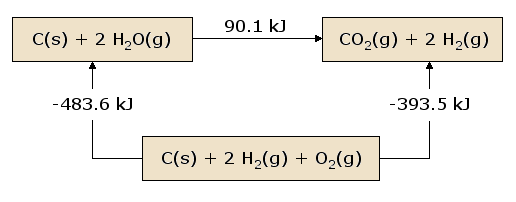# Given the following data, what is the enthalpy associated with the given equation? 

## Given... $2 {H}_{2} \left(g\right) + {O}_{2} \left(g\right) \rightarrow 2 {H}_{2} O \left(g\right)$ ;DeltaH_1^@=-484*kJ*mol^-1 $\left(i\right)$ $2 C O \left(g\right) + {O}_{2} \left(g\right) \rightarrow 2 C {O}_{2} \left(g\right)$ ;DeltaH_2^@=-566*kJ*mol^-1 $\left(i i\right)$ ...what is the enthalpy associated with the reaction...? ${H}_{2} \left(g\right) + C {O}_{2} \left(g\right) \rightarrow C O \left(g\right) + {H}_{2} O \left(g\right)$?

Apr 13, 2017

We treat the individual equations as simultaneous linear equations....
and get an endothermic reaction..........

#### Explanation:

We have:

$2 {H}_{2} \left(g\right) + {O}_{2} \left(g\right) \rightarrow 2 {H}_{2} O \left(g\right)$ ;DeltaH_1^@=-484*kJ*mol^-1 $\left(i\right)$

$2 C O \left(g\right) + {O}_{2} \left(g\right) \rightarrow 2 C {O}_{2} \left(g\right)$ ;DeltaH_2^@=-566*kJ*mol^-1 $\left(i i\right)$

We want $\Delta {H}_{\text{rxn}}^{\circ}$ for..........

$C {O}_{2} \left(g\right) + {H}_{2} \left(g\right) \rightarrow C O \left(g\right) + {H}_{2} O \left(g\right)$ ;DeltaH^@=?? $\left(i i i\right)$

If I add $\left(i\right) - \left(i i\right)$, I get...........

$2 {H}_{2} \left(g\right) + \cancel{{O}_{2} \left(g\right)} + 2 C {O}_{2} \left(g\right) \rightarrow 2 C O \left(g\right) + \cancel{{O}_{2} \left(g\right)} + 2 {H}_{2} O \left(g\right)$

$= 2 {H}_{2} \left(g\right) + 2 C {O}_{2} \left(g\right) \rightarrow 2 C O \left(g\right) + 2 {H}_{2} O \left(g\right)$

But this is EQUAL to $2 \times \left(i i i\right)$

And thus $\Delta {H}_{\text{rxn}}^{\circ} = \frac{1}{2} \left(\Delta {H}_{1}^{\circ} - \Delta {H}_{2}^{\circ}\right)$

$\frac{1}{2} \left(- 484 - \left(- 566\right)\right) \cdot k J \cdot m o {l}^{-} 1 = + 41 \cdot k J \cdot m o {l}^{-} 1$....if I have done my 'rithmetic rite!

Apr 13, 2017

This is the Hess's law

In the Hess's law you should add both the reactions like this

For exampleHess's law states that the addition of total heat absorbed and and released of some reactions is equal to$\Delta H$of the combined equation in one or many steps.This applies to the first law of thermodynamics,principle of conservation of energy
And you should know that the energy absorbed or released is expressed as $\Delta H$(enthalpy)

For an equation like

A + B=AB
C+B= CB

A + B = AB
C+ B= CB

Cancel the same reactants on both sides and add the reaction

A + C = CB + AB

Even though its not possible

So if we add the the $\Delta H$ of the reactions we can get the $\Delta H$ of this reaction

Even you can get the $\Delta H$ of sensible reaction by reversing the $\Delta H$ of a more sensible reaction

For example if the $D \eta H$ of this reaction is

C + B = CB $\Delta H$ = x
CB = C +B $\Delta H = - x$
And consider
A + B$\Delta H = d$

Now again do what we did before

A + B = AB
CB = C + B

CB + A = C + AB

And now add the $\Delta H$ of this reaction is $- x + d$

Do the same for this reaction

$2 {H}_{2} + {O}_{2} \rightarrow 2 {H}_{2} O$
$2 C O + {O}_{2} \rightarrow 2 C {O}_{2}$

If we cancel the same terms on both side that is ${O}_{2}$ and add the reactions we get

$2 {H}_{2} + 2 C O = 2 {H}_{2} O + 2 C {O}_{2}$

And that's not a sensible reaction and what we wanted
so we do what we did in the above example

$2 {H}_{2} + {O}_{2} \rightarrow 2 {H}_{2} O \textcolor{w h i t e}{X X X} \Delta H = \text{-484kJ/mol}$
 2CO(g) + O_2(g) -> 2CO_2(g) ∆H = -566 kj/mol
$2 C {O}_{2} \left(g\right) \rightarrow 2 C O \left(g\right) + {O}_{2} \left(g\right) \textcolor{w h i t e}{X X X} \Delta H = \text{-("-566 kj/mol") = "566kJ/mol}$

$2 C {O}_{2} \left(g\right) + 2 {H}_{2} \left(g\right) + {O}_{2} \left(g\right) \to 2 C O \left(g\right) + 2 {H}_{2} O \left(g\right) + {O}_{2} \left(g\right)$

Cancel the same terms

$2 C {O}_{2} \left(g\right) + 2 {H}_{2} \left(g\right) + \cancel{{O}_{2} \left(g\right)} \to 2 C O \left(g\right) + 2 {H}_{2} O \left(g\right) + \cancel{{O}_{2} \left(g\right)}$

Now add the $\Delta H$

$- 484 k \frac{J}{m} o l + 566 k \frac{J}{m} o l = 82 k \frac{J}{m} o l$

But this is the $\Delta H$ of

$2 C {O}_{2} \left(g\right) + 2 {H}_{2} \left(g\right) \to 2 C O \left(g\right) + 2 {H}_{2} O \left(g\right)$

not

$C {O}_{2} \left(g\right) + {H}_{2} \left(g\right) \to C O \left(g\right) + {H}_{2} O \left(g\right)$

To caculate the $\Delta H$of this reaction divide the $\Delta H$ by two

= 41kJ/mol